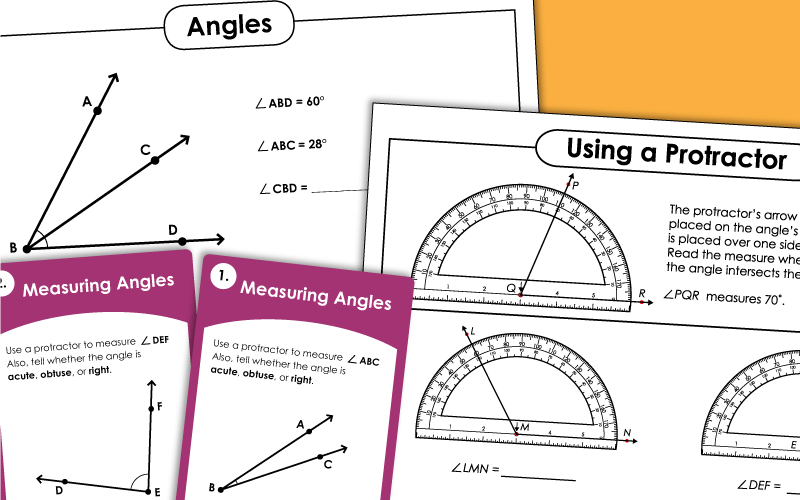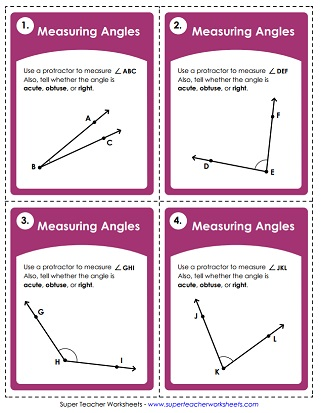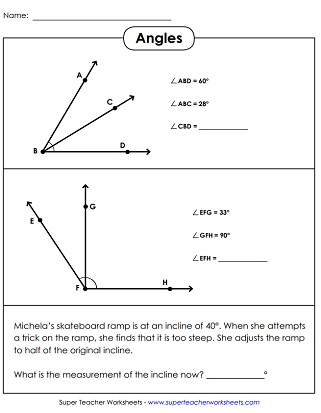# Measuring Angles

These printable geometry worksheets will help students learn to measure angles with a protractor, and draw angles with a given measurement.

Visit the Types of Angles page for worksheets on identifying acute, right, and obtuse angles.## Measuring AnglesWorksheets

Measure each the angles with a protractor.  Angles spun in all different directions.
On these task cards, students will use their protractor to measure angles. File includes 30 cards and an answer page.
Measure the angles using the protractor.  All angles face right.
Measure the angles using the protractor.  Angles face right and left.
Use a protractor to measure the angles.  All angles face right.
Use a protractor to measure each of these angles.  Angles face right and left.
Measure each of these angles with a protractor.  All angles face right.
Measure each of these angles with a protractor.  Includes both left and right facing angles.

This worksheet has pictures of pairs of adjacent angles. Students should recognize them as additive and find the measurement of the missing angle.

## Printable Protractor

Need protractors for your students? Print this page on heavy card stock. Students can cut them out and use them to measure and draw angles.
Angles (Types: Acute, Obtuse, Right)

This page has printables to help students identify types of angles. Most cover acute, obtuse, and right angles. A few also cover straight and reflex angles.

Complementary and Supplementary Angles

This page has worksheets for teaching students about complementary angles (angles whose measurements add up to 90 degrees) and supplementary angles (whose measurements add up to 180 degrees).

Geometry Worksheets

Shapes, lines, perimeter, area, symmetry, radius/diameter, and flips, slides, turns, and more.

## Pictures of Our PrintablesMy Account
Site Information